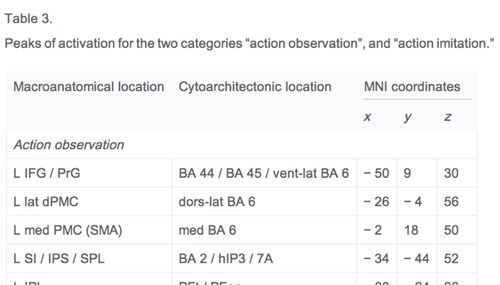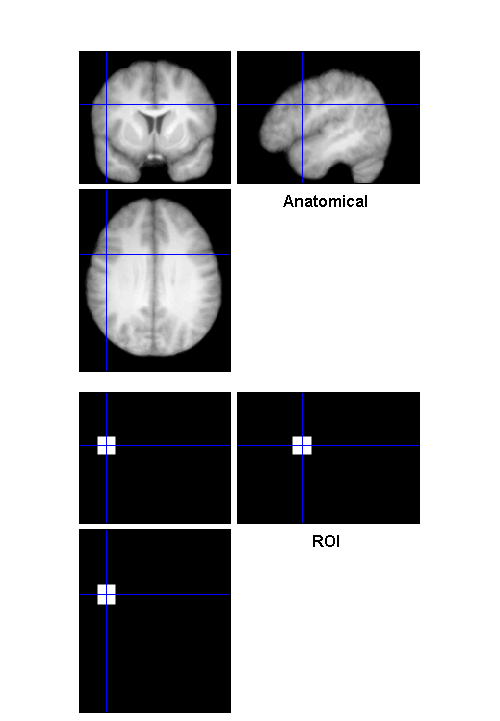## Making ROIs from Activation Peak Coordinates

Imagine you study the neural bases of action understanding, and you want to test hypotheses about regions that have been consistently implicated in previous neuroimaging studies of action understanding. You notice this meta-analysis in NeuroImage:You read the paper and find Table 3, which contains a bunch of meta-analytically defined coordinates:Wouldn't it be nice to easily convert those coordinates into ROI images that you can use in your own research? Here's a way:

## Use the HELP function to see documentation for using "bspm_coords2roi"

```help bspm_coords2roi
```
```  BSPM_COORDS2ROI

USAGE: bspm_coords2roi(ref,xyz,roi)

ARGUMENTS
xyz = coordinates (rows are ROIs)
roi.shape = 'Sphere' or 'Box'
roi.size = radius of ROI

Uses code authored by:
Dr. Robert Welsh (SimpleROIBuilder.m)
Drs. Donald McLaren & Aaron Schultz (peak_nii.m)

```

## Define the inputs

```xyz         = [-50 9 30];   % the XYZ coordinate from the top of Table 3
roi.shape   = 'Sphere';     % Box is an option too
roi.size    = 9;            % radius of our sphere (millimeters)
labels       = 'L_IFG';     % label for the region (for the filename)
```

## Run it

```bspm_coords2roi(xyz, roi, labels);
```
```ROI file created: ROI_Sphere9_-50_9_30_L_IFG.nii
```

## Check to make sure it looks like left inferior frontal gyrus

```anatimg = 'mean_wanat_hires.nii';
roiimg = 'ROI_Sphere9_-50_9_30_L_IFG.nii';
bspm_checkreg({anatimg; roiimg}, {'Anatomical' 'ROI'}, xyz);
```## Here's what a box looks like. A BIG box.

```xyz         = [-50 9 30];   % the XYZ coordinate from the top of Table 3
roi.shape   = 'Box';     % Box is an option too
roi.size    = 18;            % radius of our sphere (millimeters)
labels       = 'L_IFG';     % label for the region (for the filename)
```

## Run it

```bspm_coords2roi(xyz, roi, labels);
```
```ROI file created: ROI_Box18_-50_9_30_L_IFG.nii
```

## Check to make sure it looks like left inferior frontal gyrus

```anatimg = 'mean_wanat_hires.nii';
roiimg = 'ROI_Box18_-50_9_30_L_IFG.nii';
bspm_checkreg({anatimg; roiimg}, {'Anatomical' 'ROI'}, xyz);
```